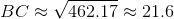# SAT II Math II : Law of Cosines

## Example Questions

### Example Question #1 : Law Of Cosines

A triangle has sides that measure 10, 12, and 16. What is the greatest measure of any of its angles (nearest tenth of a degree)?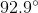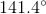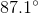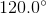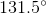Explanation:

We are seeking the measure of the angle opposite the side of greatest length, 16.

We can use the Law of Cosines, setting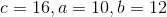, and solving for: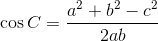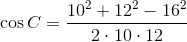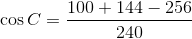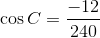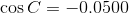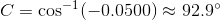### Example Question #1 : Law Of Cosines

A triangle has sides that measure 15, 17, and 30. What is the least measure of any of its angles (nearest tenth of a degree)?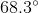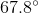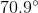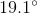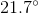Explanation:

We are seeking the measure of the angle opposite the side of least length, 15.

We can use the Law of Cosines, setting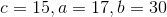, and solving for: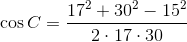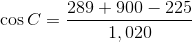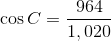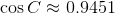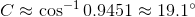### Example Question #3 : Law Of Cosines

Given :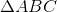with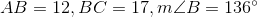.

Which of the following whole numbers is closest to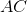?Explanation:

Apply the Law of Cosines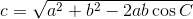setting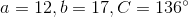and solving for: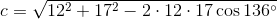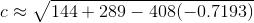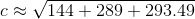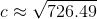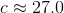Of the five choices, 27 comes closest.

### Example Question #4 : Law Of Cosines

Given :with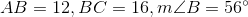.

Evaluateto the nearest tenth.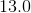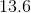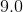The correct answer is not given among the other responses.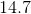Explanation:

Apply the Law of Cosinessetting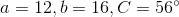and solving for: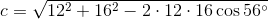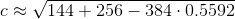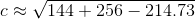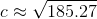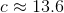### Example Question #5 : Law Of Cosines

In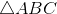: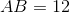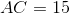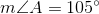Evaluate the length of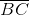to the nearest tenth of a unit.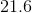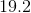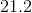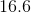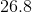Explanation:

The figure referenced is below: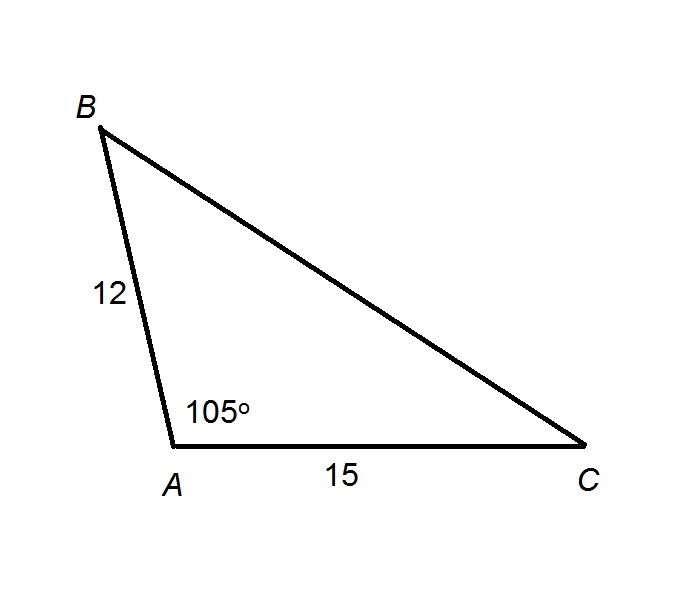By the Law of Cosines, given the lengthsandof two sides of a triangle, and the measure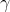of their included angle, the lengthof the third side can be calculated using the formula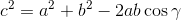Substituting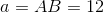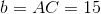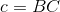, and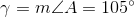, then evaluating: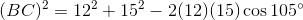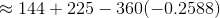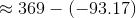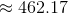Taking the square root of both sides: